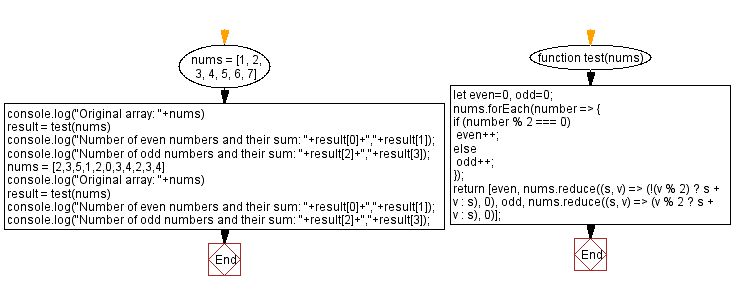# JavaScript: Even, odd numbers and their sum in an array

## JavaScript Math: Exercise-94 with Solution

Write a JavaScript program to calculate the sum and count of even and odd numbers in an array.

Test Data:
([1,2,3,4,5,6,7]) -> 3,12, 4,16
([2,3,5,1,2,0,3,4,2,3,4)] -> 6,14, 5,15

Sample Solution:

HTML Code:

``````<!DOCTYPE html>
<html>
<meta charset="utf-8">
<title>JavaScript program to Even, odd numbers and their sum in an array</title>
<body>

</body>
</html>
```
```

JavaScript Code:

``````function test(nums) {
let even=0, odd=0;
nums.forEach(number => {
if (number % 2 === 0)
even++;
else
odd++;
});
return [even,
nums.reduce((s, v) => (!(v % 2) ? s + v : s), 0), odd,
nums.reduce((s, v) => (v % 2 ? s + v : s), 0)];
}

nums = [1, 2, 3, 4, 5, 6, 7]
console.log("Original array: "+nums)
result = test(nums)
console.log("Number of even numbers and their sum: "+result+","+result);
console.log("Number of odd numbers and their sum: "+result+","+result);
nums = [2,3,5,1,2,0,3,4,2,3,4]
console.log("Original array: "+nums)
result = test(nums)
console.log("Number of even numbers and their sum: "+result+","+result);
console.log("Number of odd numbers and their sum: "+result+","+result);
```
```

Sample Output:

```Original array: 1,2,3,4,5,6,7
Number of even numbers and their sum: 3,12
Number of odd numbers and their sum: 4,16
Original array: 2,3,5,1,2,0,3,4,2,3,4
Number of even numbers and their sum: 6,14
Number of odd numbers and their sum: 5,15
```

Flowchart:Live Demo:

See the Pen javascript-math-exercise-94 by w3resource (@w3resource) on CodePen.

Improve this sample solution and post your code through Disqus

What is the difficulty level of this exercise?

Test your Programming skills with w3resource's quiz.

﻿